#Transum Trigonometry is a collection of online exercises. The questions are presented in different formats to deepen understanding. The first collection (Basic) contains 8 levels question with most of the numbers randomly generated. Collections 1 and 2 are self checking. When you have answered enough questions you are given the option of claiming a virtual Transum trophy showing how many questions you have answered correctly. It is recommended that you write out all of your working in your exercise book and then check your final answser online. Answers should be given to three significant figures unless otherwise stated. To get started click on one of the images below. Good luck!

#### Basic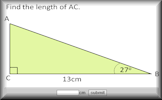Basic questions involving the sine, cosine and tangent ratios. Either a side or an angle are to be found.

Start

#### Three Dimensional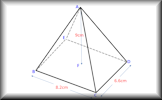More advanced problem solving techniques are needed to answer these 3D questions.

Start
##### Collection 3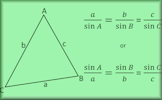A self-marking exercise on the sine rule, cosine rule and the sine formula for finding the area of a triangle.

Start

#### Which Side?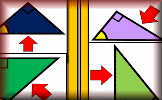Arrange the diagrams in groups depending on whether the arrow is pointing to the opposite, adjacent or hypotenuse.

Start

#### 3D Trig PresentationA slide presentation introducing using trigonometry (including Pythagoras' Theorem) to find lengths and angles on three dimensional shapes.

Start

#### Pythagoras BasicsA drill and practice self marking exercise on the basics of Pythagoras' theorem. There are a number of levels including mixed questions.

Start

#### Exam Style Questions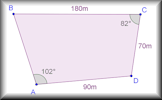GCSE and IB past paper style questions which may include the sine and cosine rules. Work solutions are available.

Start

#### Common Trig Ratios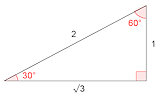A self-marking exercise on finding the exact values of sine, cosine and tangent of special angles.

Start

#### Inverse Calculator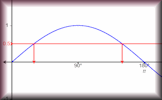This calculator is designed to find all of the angles for a given trigonometric ratio and show them on a graph.

Start

The solutions to these and other Transum puzzles, exercises and activities are available here when you are signed in to your Transum subscription account. If you do not yet have an account and you are a teacher or parent you can apply for one here.

You can find links to a Trigonometry Starter, investigation, videos and external sites on the main Trigonometry topic page.

Frances, Munich

Tuesday, May 15, 2018

"Hello, I LOVE your website it is so useful!!!!"

John Rodger,

Thursday, November 17, 2022

"Hi, I have looked for something that specifically looks at angles of elevation and depression. Unable to find these as a separate of questions. Do you have a specific of questions relating to this?
Many thanks.

[Transum: Thanks for your comment John. You can find exam-style questions on the Angles of Elevation and Depression page.]"

Do you have any comments? It is always useful to receive feedback and helps make this free resource even more useful for those learning Mathematics anywhere in the world. Click here to enter your comments.For All: